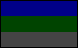This document is part of the Martian Time Boneyard. It was originally located at http://www.geocities.com/sotosoroto/modvoph.html. Author: Mark KnokePossible Perpetual Calendars for Mars

Mark Knoke

Aren't prime numbers just grand? I've been working with numbers between 658 and 679, finding their primes. For the following list, I threw out combinations with prime numbers over 20 and I kept the number of months above 9 and below the length of the month. In parentheses are possible perpetual week-lengths.

19 of 35 (5)(7)

17 of 39 (13)

10 of 66 (6)(11)
11 of 60 (5)(6)(10)(12)(15)
12 of 55 (5)(11)
15 of 44 (11)
20 of 33 (11)
22 of 30 (5)(6)(10)

Subtract 3.5 days:

12 of 56 (7)(8)
14 of 48 (6)(8)(12)
16 of 42 (6)(7)
21 of 32 (8)
24 of 28 (7)

Subtract 6.5 days:

9 of 75 (5)(15)
15 of 45 (5)(9)(15)
25 of 27 (9)

Subtract 7.5 days:

26 of 26 (13)

...So which'll it be, oh fans of ultra-perpetualism?

The Primes:

658 = 2 x 7 x 47
659 = 659
660 = 2 x 2 x 3 x 5 x 11
661 = 661
662 = 2 x 331
663 = 3 x 13 x 17
664 = 2 x 2 x 2 x 83
665 = 5 x 7 x 19
666 = 2 x 3 x 3 x 37
667 = 23 x 29
668 = 2 x 2 x 167
669 = 3 x 223
670 = 2 x 5 x 67
671 = 11 x 61
672 = 2 x 2 x 2 x 2 x 2 x 3 x 7
673 = 673
674 = 2 x 337
675 = 3 x 3 x 3 x 5 x 5
676 = 2 x 2 x 13 x 13
677 = 677
678 = 2 x 3 x 113
679 = 7 x 97. . | . .. . | . .to my homepage . | . to my Mars page . | . to my Narakan page